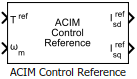# ACIM Control Reference

Compute reference currents for field-oriented control of induction motor

• Library:
• Motor Control Blockset / Controls / Control Reference

•## Description

The ACIM Control Reference block computes the d-axis and q-axis reference currents for the field-oriented control (and field-weakening) operation.

The block accepts the reference torque and feedback mechanical speed and outputs the corresponding d- and q-axes reference currents.

The block computes the reference current values by solving mathematical relationships. The calculations use the SI unit system. When working with the Per-Unit (PU) system (with the Input units parameter set to `Per-Unit (PU)`), the block converts PU input signals to SI units to perform the computations and converts them back to PU values at the output.

These equations describe how the block computes the reference d-axis and q-axis current values.

### Mathematical Model of Induction Motor

These model equations describe the dynamics of induction motor in the rotor flux reference frame:

The machine inductances are represented as,

`$\sigma =1-\left(\frac{{L}_{m}^{2}}{{L}_{s}\cdot {L}_{r}}\right)$`

Stator voltages are represented as,

In the preceding equations, the flux linkages can be represented as,

If we keep the rotor flux as constant and the d-axis is aligned to the rotor flux reference frame, then we can imply:

`${\lambda }_{\text{rd}}={L}_{m}{i}_{sd}$`
`${\lambda }_{\text{rq}}=0$`

These equations describe the mechanical dynamics,

`${T}_{e}=\frac{3}{2}p\left(\frac{{L}_{m}}{{L}_{r}}\right){\lambda }_{rd}{i}_{sq}$`

These equations describe the slip speed,

### Reference Current Computation

These equations show computation of the reference currents,

`${i}_{sd_0}=\frac{{\lambda }_{\text{rd}}}{{\text{L}}_{\text{m}}}$`

The reference currents are computed differently for operation below base speed and field weakening region,

If :

`${i}_{sd_sat}=\mathrm{min}\left({i}_{sd_0},{i}_{\mathrm{max}}\right)$`

If :

`${i}_{sd_fw}={i}_{sd_0}\left(\frac{{\omega }_{rated}}{{\omega }_{m}}\right)$`
`${i}_{sd_sat}=\mathrm{min}\left({i}_{sd_fw},{i}_{\mathrm{max}}\right)$`

These equations indicate the q-axis current computation,

`${i}_{sq_sat}=sat\left({i}_{sq_lim},{i}_{sq_req}\right)$`

The block outputs the following values,

`${i}_{sd}^{ref}={i}_{sd_sat}$`
`${i}_{sq}^{ref}={i}_{sq_sat}$`

where:

• $p$ is the number of pole pairs of the motor.

• ${R}_{s}$ is the stator phase winding resistance (Ohms).

• ${R}_{r}$ is the rotor resistance referred to stator (Ohms).

• ${L}_{ls}$ is the stator leakage inductance (Henry).

• ${L}_{lr}$ is the rotor leakage inductance (Henry).

• ${L}_{s}$ is the stator inductance (Henry).

• ${L}_{m}$ is the magnetizing inductance (Henry).

• ${L}_{r}$ is the rotor inductance referred to stator (Henry).

• $\sigma$ is the total leakage factor of the induction motor.

• ${\tau }_{\text{r}}$ is the rotor time constant (sec).

• ${v}_{sd}$ and ${v}_{sq}$ are the stator d- and q-axis voltages (Volts).

• ${i}_{sd}$ and ${i}_{sq}$ are the stator d- and q-axis currents (Amperes).

• ${i}_{sd_0}$ is the rated d-axis current of the stator also known as magnetizing current (Amperes).

• ${i}_{max}$ is the maximum phase current (peak) of the motor (Amperes).

• ${\lambda }_{sd}$ is the d-axis flux linkage of the stator (Weber).

• ${\lambda }_{sq}$ is the q-axis flux linkage of the stator (Weber).

• ${\lambda }_{rd}$ is the d-axis flux linkage of the rotor (Weber).

• ${\lambda }_{rq}$ is the q-axis flux linkage of the rotor (Weber).

• ${\omega }_{\text{e_slip}}$ is the electrical slip speed of the rotor (Radians/ sec).

• ${\omega }_{\text{slip}}$ is the mechanical slip speed of the rotor (Radians/ sec).

• ${\omega }_{e}$ is the electrical speed corresponding to frequency of stator voltages (Radians/ sec).

• ${\omega }_{m}$ is the rotor mechanical speed (Radians/ sec).

• ${\omega }_{r}$ is the rotor electrical speed (Radians/ sec).

• ${\omega }_{rated}$ is the rated mechanical speed of the motor (Radians/ sec).

• ${T}_{e}$ is the electromechanical torque produced by the motor (Nm).

## Ports

### Input

expand all

Reference torque input value for which the block computes the reference current.

Data Types: `single` | `double` | `fixed point`

Reference mechanical speed value for which the block computes the reference current.

Data Types: `single` | `double` | `fixed point`

### Output

expand all

Reference d-axis stator current value.

Data Types: `single` | `double` | `fixed point`

Reference q-axis stator current value.

Data Types: `single` | `double` | `fixed point`

## Parameters

expand all

Number of pole pairs available in the induction motor.

Inductance due to leakage flux linked to the rotor winding (in Henry).

Inductance due to the magnetizing flux (in Henry).

Rated flux of the induction motor (in Weber).

Rated speed of the induction motor according to motor data sheet (in rpm).

Synchronous speed of the induction motor (in rpm).

Maximum phase current limit for the induction motor (amperes).

Unit of the input values.

Base current (in Amperes) for per-unit system.

#### Dependencies

To enable this parameter, set Input units to `Per-Unit (PU)`.

Base torque (in Nm) for per-unit system. See Per-Unit System page for more details.

This parameter is not configurable and uses a value that is internally computed using other parameters.

#### Dependencies

To display this parameter, set Input units to `Per-Unit (PU)`.

 B. Bose, Modern Power Electronics and AC Drives. Prentice Hall, 2001. ISBN-0-13-016743-6.

 Lorenz, Robert D., Thomas Lipo, and Donald W. Novotny. "Motion control with induction motors." Proceedings of the IEEE, Vol. 82, Issue 8, August 1994, pp. 1215-1240.

 W. Leonhard, Control of Electrical Drives, 3rd ed. Secaucus, NJ, USA:Springer-Verlag New York, Inc., 2001.

 Briz, Fernando, Michael W. Degner, and Robert D. Lorenz. "Analysis and design of current regulators using complex vectors." IEEE Transactions on Industry Applications, Vol. 36, Issue 3, May/June 2000, pp. 817-825.

 Briz, Fernando, et al. "Current and flux regulation in field-weakening operation [of induction motors]." IEEE Transactions on Industry Applications, Vol. 37, Issue 1, Jan/Feb 2001, pp. 42-50.

 R. M. Prasad and M. A. Mulla, “A novel position-sensorless algorithm for field oriented control of DFIG with reduced current sensors,” IEEE Trans. Sustain. Energy, vol. 10, no. 3, pp. 1098–1108, July 2019.